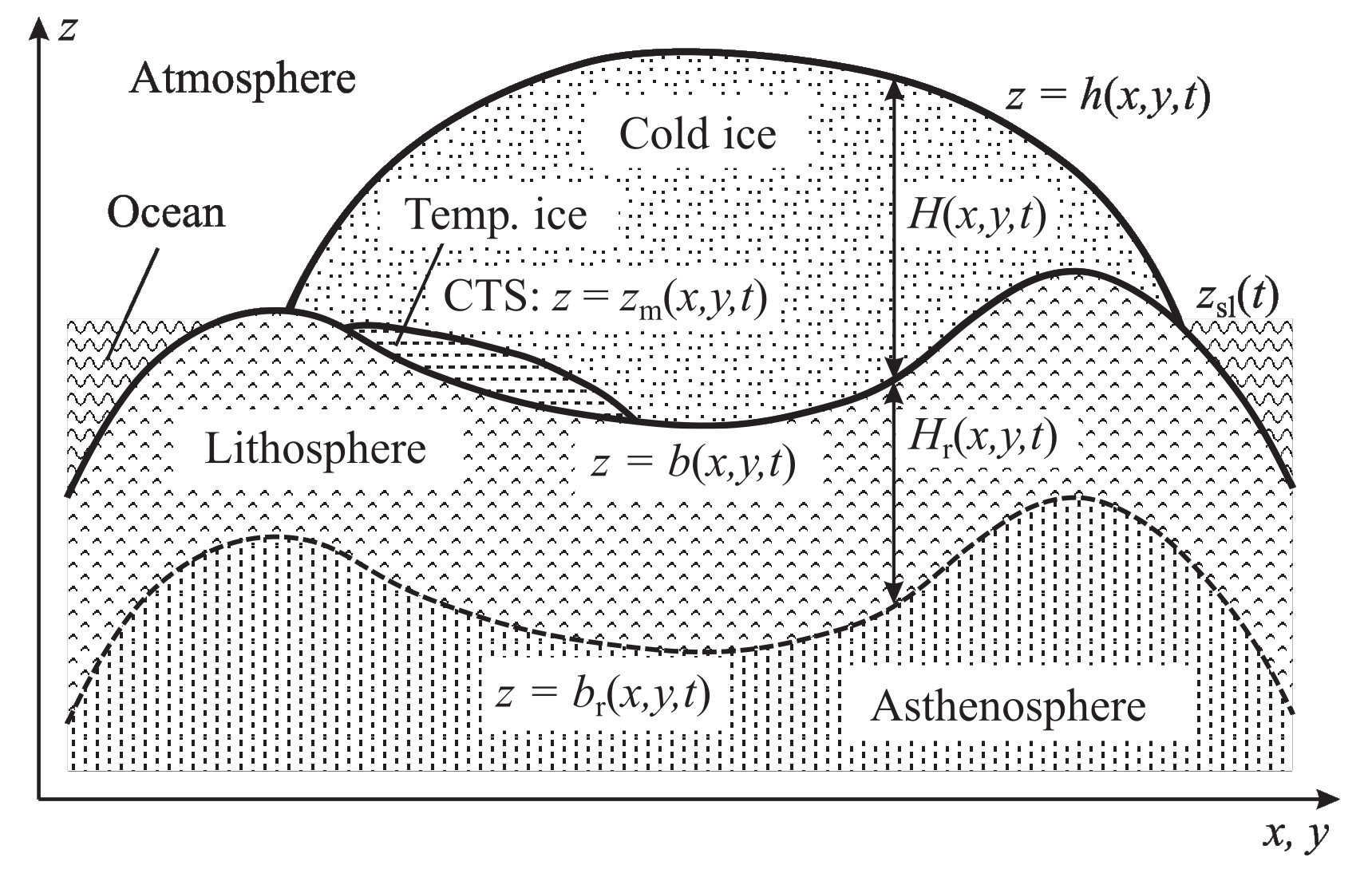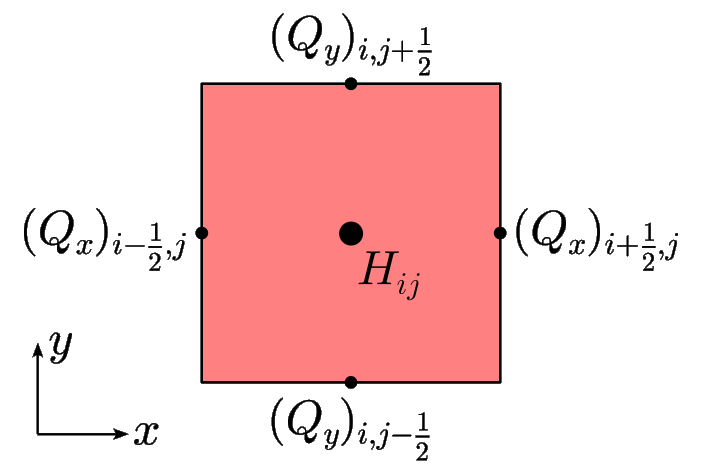# 6.1. Model domain, grid and time¶

## 6.1.1. Model domain¶

### 6.1.1.1. Selecting a pre-defined domain¶

SICOPOLIS provides several pre-defined model domains. They can be chosen by defining a domain code in the run-specs header as follows:

#define GRL
!             Simulated domain:
!               ANT     - Antarctica
!               ASF     - Austfonna
!               EISMINT - EISMINT (Phase 2 SGE and modifications)
!               GRL     - Greenland
!               NHEM    - Northern hemisphere
!               SCAND   - Scandinavia
!               TIBET   - Tibet
!               NMARS   - North polar cap of Mars
!               SMARS   - South polar cap of Mars


This example would select the domain for the Greenland ice sheet. Correspondingly for the other listed domains.

### 6.1.1.2. Setting up a new domain¶

In addition to the pre-defined domains, there is an unspecified domain XYZ. This framework allows creating new domains (Laurentide ice sheet, some ice cap, simple testing geometry…) quite easily. The directory src/subroutines/xyz, which hosts the domain-specific subroutines, is by default empty. If you want to create a new domain, copy the subroutines from the most similar existing domain, e.g., starting from Antarctica:

cp src/subroutines/ant/*.F90 src/subroutines/xyz/


Then modify the routines according to your needs. Input files (topography etc.) must be placed in sico_in/xyz and specified in the run-specs header file *.h as usual. The domain must be defined by the domain code

#define XYZ


in the header. For flexible testing, it is recommended to deactivate the compatibility check between horizontal resolution and number of grid points:

#define CHECK_RES_IMAX_JMAX 0


If the new domain requires new global variables, they can be defined in the module src/subroutines/xyz/sico_vars.F90.

The subroutines for ISMIP HEINO (Calov et al. ) are available in src/subroutines/xyz/heino, and the input files are in sico_in/xyz. If you copy the subroutines from src/subroutines/xyz/heino to src/subroutines/xyz, you can run ISMIP HEINO experiments (e.g., the run repo_heino50_st for which a run-specs header file is available).

## 6.1.2. Spatial grid¶

In principle, SICOPOLIS allows using any orthogonal coordinates on the Earth’s surface, provided that the two components $$g_{11}$$ and $$g_{22}$$ of the metric tensor are known (see “Orthogonal coordinates on the Earth’s surface”). They are computed in the module metric_m. Three options are currently implemented and can be selected in the run-specs header by the parameter GRID:

• 0: Cartesian coordinates in the stereographic plane without distortion correction.

• 1: Cartesian coordinates in the stereographic plane with distortion correction.

• 2: Geographical coordinates (longitude/latitude).

For the most common case of Cartesian coordinates $$x$$ and $$y$$ in the stereographic plane (or any other projection plane), let the domain be the rectangle described by $$[x_0,x_\mathrm{max}]$$, $$[y_0,y_\mathrm{max}]$$. It is discretized by a regular (structured) grid with horizontal resolution $$\Delta{x}$$, which is the same for the $$x$$- and $$y$$-directions. The location of the grid points $$x_i$$ and $$y_j$$ is then given by

(6.1)$x_i = x_0 + i\Delta{x}, \qquad i=0\,(1)\,i_\mathrm{max},$
(6.2)$y_j = y_0 + j\Delta{x}, \qquad j=0\,(1)\,j_\mathrm{max},$

where the notation $$a\,(b)\,c$$ means “from $$a$$ to $$c$$ in steps of $$b$$”. Note that the indices $$i$$ and $$j$$ run from 0, so that the number of grid points is actually $$i_\mathrm{max}+1$$ and $$j_\mathrm{max}+1$$, respectively. In the run-specs headers, the parameters to be defined are

• X0 ($$=x_0$$, $$x$$ coordinate of the origin point in km),

• Y0 ($$=y_0$$, $$y$$ coordinate of the origin point in km),

• DX ($$=\Delta{}x$$, horizontal grid spacing in km),

• IMAX ($$=i_\mathrm{max}$$, maximum value of the index $$i$$),

• JMAX ($$=j_\mathrm{max}$$, maximum value of the index $$j$$).

For the vertical ($$z$$) direction, a terrain-following (“sigma”) transformation is employed that maps vertical columns in the physical space onto $$[0,1]$$ intervals. If the polythermal two-layer method (POLY, see Section “Ice thermodynamics”) is employed, this mapping is done separately for the upper cold-ice layer ($$\zeta_\mathrm{c}$$ domain), the lower temperate-ice layer ($$\zeta_\mathrm{t}$$ domain) and the lithosphere layer ($$\zeta_\mathrm{r}$$ domain). The transformation is linear for the $$\zeta_\mathrm{t}$$ and $$\zeta_\mathrm{r}$$ domains. However, for the $$\zeta_\mathrm{c}$$ domain, exponential stretching is used so that equidistant grid points in the transformed domain map on grid points concentrating towards the base in the physical $$z$$-coordinate:

(6.3)$\frac{z-z_\mathrm{m}}{H_\mathrm{c}} = \frac{e^{a\zeta_\mathrm{c}}-1}{e^a-1}, \qquad \frac{z-b}{H_\mathrm{t}} = \zeta_\mathrm{t}, \qquad \frac{z-b_\mathrm{r}}{H_\mathrm{r}} = \zeta_\mathrm{r},$

where the geometric quantities are explained in Fig. 6.1 and $$a$$ is the exponential stretch parameter for the $$\zeta_\mathrm{c}$$ domain. For this parameter, $$a=2$$ is a typical choice, while the limit $$a=0$$ produces a linear transformation.Fig. 6.1 Cross section through a polythermal ice sheet (vertically exaggerated).¶ $$h$$: position of the ice surface, $$z_\mathrm{m}$$: position of the CTS (CTS: “cold-temperate transition surface”, interface between the cold-ice and temperate-ice layers), $$b$$: position of the ice base, $$b_\mathrm{r}$$: position of the base of the lithosphere layer, $$H=h-b$$: ice thickness, $$H_\mathrm{c}=h-z_\mathrm{m}$$: thickness of the cold-ice layer, $$H_\mathrm{t}=z_\mathrm{m}-b$$: thickness of the temperate-ice layer, if existing (thus $$H=H_\mathrm{c}+H_\mathrm{t}$$), $$H_\mathrm{r}=b-b_\mathrm{r}$$: thickness of the lithosphere (rock) layer.

The location of the grid points in the three transformed domains is given by

(6.4)$(\zeta_\mathrm{c})_{k_\mathrm{c}} = k_\mathrm{c}/k_\mathrm{c,max}, \qquad k_\mathrm{c}=0\,(1)\,k_\mathrm{c,max},$
(6.5)$(\zeta_\mathrm{t})_{k_\mathrm{t}} = k_\mathrm{t}/k_\mathrm{t,max}, \qquad k_\mathrm{t}=0\,(1)\,k_\mathrm{t,max},$
(6.6)$(\zeta_\mathrm{r})_{k_\mathrm{r}} = k_\mathrm{r}/k_\mathrm{r,max}, \qquad k_\mathrm{r}=0\,(1)\,k_\mathrm{r,max}.$

The numbers of grid points result as $$k_\mathrm{c,max}+1$$, $$k_\mathrm{t,max}+1$$ and $$k_\mathrm{r,max}+1$$, respectively. The parameters in the run-specs headers are

• KCMAX ($$=k_\mathrm{c,max}$$, maximum value of the index $$k_\mathrm{c}$$),

• KTMAX ($$=k_\mathrm{t,max}$$, maximum value of the index $$k_\mathrm{t}$$),

• KRMAX ($$=k_\mathrm{r,max}$$, maximum value of the index $$k_\mathrm{r}$$),

• DEFORM ($$=a$$, exponential stretch parameter for the $$\zeta_\mathrm{c}$$ domain).

For all other thermodynamics schemes (ENTC, ENTM, COLD, ISOT; see Section “Ice thermodynamics”), the entire ice column (no matter whether cold or temperate) is mapped on the $$\zeta_\mathrm{c}$$ domain. The $$\zeta_\mathrm{t}$$ domain is then redundant and collapses onto the ice base:

(6.7)$\frac{z-b}{H} = \frac{e^{a\zeta_\mathrm{c}}-1}{e^a-1}, \qquad b = \zeta_\mathrm{t}, \qquad \frac{z-b_\mathrm{r}}{H_\mathrm{r}} = \zeta_\mathrm{r}.$

For technical reasons, the $$\zeta_\mathrm{t}$$ domain is still present and should be assigned three grid points, that is, KTMAX should be set to 2.

A staggered Arakawa C grid is used for reasons of numerical stability (Arakawa and Lamb ). This means that the components of the velocity ($$v_{x}$$, $$v_{y}$$, $$v_{z}$$) and the volume flux (depth-integrated horizontal velocity; $$Q_{x}$$, $$Q_{y}$$) are defined in between the grid points, while all other quantities are defined on the grid points.Fig. 6.2 Arakawa C grid for the ice thickness $$H$$ and the volume flux $$(Q_{x},Q_{y})$$ in one grid cell in the horizontal plane, centred around the grid point $$(i,j)$$.¶

For the example of the 2D fields ice thickness and volume flux, this is illustrated in Fig. 6.2. For the 3D fields, the principle is the same: The velocity components are defined in between the grid points:

(6.8)$(v_{x})_{i\pm\frac{1}{2},j,k} \; , \quad (v_{y})_{i,j\pm\frac{1}{2},k} \; , \quad (v_{z})_{i,j,k\pm\frac{1}{2}} \; ,$

while other fields like the temperature $$T$$ are defined on the grid points: $$T_{ijk}$$. Note that, depending on the layer ($$\zeta_\mathrm{c,t,r}$$ domains, see above), the index $$k$$ can either be $$k_\mathrm{c}$$, $$k_\mathrm{t}$$ or $$k_\mathrm{r}$$.

Since half-integer indices are not allowed in Fortran, they are rounded down in the code. For the example of the velocity component $$v_{x}$$ in the $$k_\mathrm{c}$$ domain:

(6.9)$(v_{x})_{i+\frac{1}{2},j,k_\mathrm{c}} \; \rightarrow \; \mbox{vx_c(kc,j,i)}\,, \quad (v_{x})_{i-\frac{1}{2},j,k_\mathrm{c}} \; \rightarrow \; \mbox{vx_c(kc,j,i-1)}\,.$

## 6.1.3. Topography¶

Gridded present-day topographies that match the horizontal grid must be provided in either NetCDF (*.nc) or ASCII (any other file extension) format. They can be specified in the run-specs header as follows (example with NetCDF files for simulation repo_grl16_bm5_ss25ka):

#define ZS_PRESENT_FILE   'grl_bm5_16_topo.nc'
!                             Name of the file containing the present-day
!                             ice-surface topography
!                             (if NetCDF, variable name must be 'zs')

#define ZB_PRESENT_FILE   'grl_bm5_16_topo.nc'
!                             Name of the file containing the present-day
!                             ice-base topography (only for ANF_DAT==1)
!                             (if NetCDF, variable name must be 'zb')

#define ZL_PRESENT_FILE   'grl_bm5_16_topo.nc'
!                             Name of the file containing the present-day
!                             lithosphere-surface topography
!                             (only for ANF_DAT==1)
!                             (if NetCDF, variable name must be 'zl')

#define ZL0_FILE          'grl_bm5_16_zl0_llra.nc'
!                             Name of the file containing the topography
!                             of the relaxed lithosphere surface
!                             (if NetCDF, variable name must be 'zl0')

!                             Name of the file containing the present-day
!                             (if NetCDF, variable name must be 'mask')

!                             Name of the file containing the region mask
!                             ('none' if no file is to be defined)
!                             (if NetCDF, variable name must be 'mask_region')


## 6.1.4. Model time¶

Model time runs from an initial time $$t_\mathrm{init}$$ until a final time $$t_\mathrm{final}$$. For the numerical solution, this interval is discretized by different time steps:

• $$\Delta{}t$$: dynamic time step, for computing velocity and topography,

• $$\Delta{}t_\mathrm{temp}$$: thermodynamic time step, for computing temperature, water content and age,

• $$\Delta{}t_\mathrm{wss}$$: isostatic time step, for computing the isostatic steady-state displacement of the lithosphere (only if the elastic-lithosphere model is chosen).

The thermodynamic and isostatic time steps must be equal to or integer multiples of the dynamic time step. The values can be specified in the run-specs header as follows:

• TIME_INIT0 ($$=t_\mathrm{init}$$, initial time, in a),

• TIME_END0 ($$=t_\mathrm{final}$$, final time, in a),

• DTIME0 ($$=\Delta{}t$$, dynamic time step, in a),

• DTIME_TEMP0 ($$=\Delta{}t_\mathrm{temp}$$, thermodynamic time step, in a),

• DTIME_WSS0 ($$=\Delta{}t_\mathrm{wss}$$, isostatic time step, in a).

Further, there is a parameter YEAR_ZERO that specifies the SICOPOLIS year zero in astronomical year numbering [= signed year CE (AD)]. For instance, if set to 1990, the time count of SICOPOLIS will be relative to the calendar year 1990 CE. TIME_INIT0 and TIME_END0 must be given in this SICOPOLIS calendar.